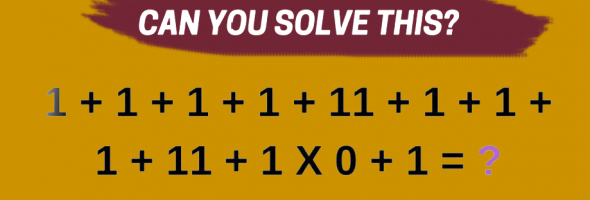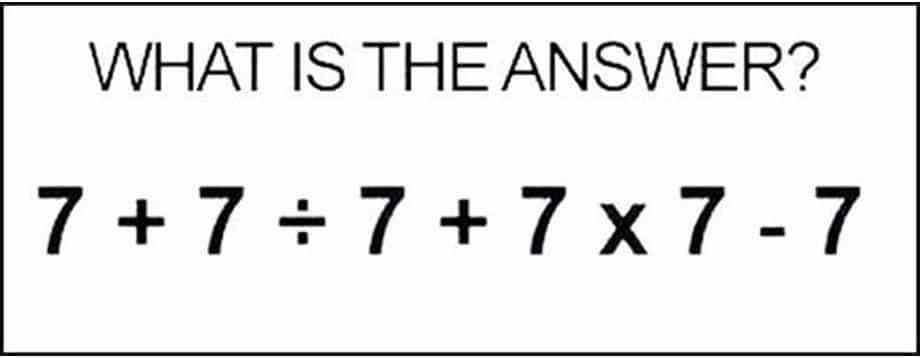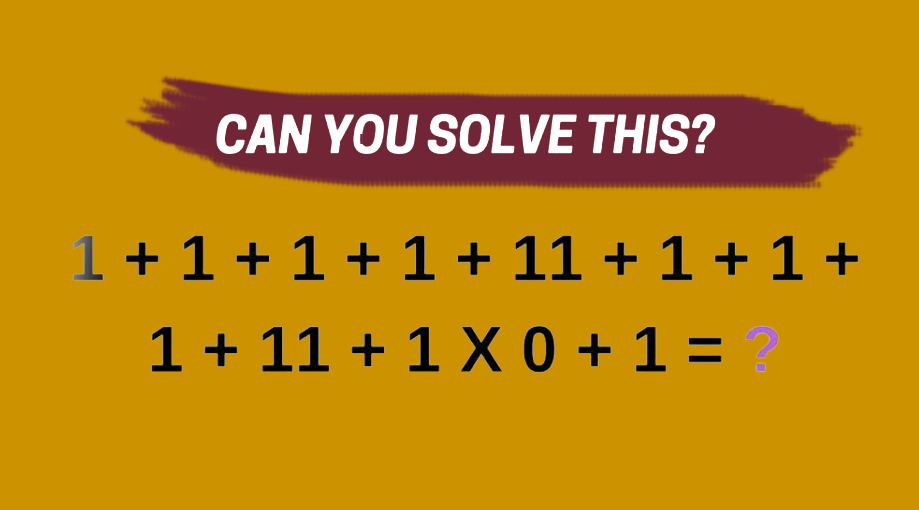## Brainteaser: Can You Solve This Simple Math Puzzle?July 3, 2019 QUIZZ

Who doesn’t love a good brainteaser? It’s a fun way to challenge your brain, which is probably why they’ve become so popular on the internet in recent months.

## Wrap your head around this math problem: 7 + 7 ÷ 7 + 7 x 7 – 7 = ?

Can you come up with the answer? If you can’t, maybe the elementary student in your life can help.Think you solved it? The answer is below.

.

.

.

.

.

It’s basic math at its finest. You might think the answer is 56, but you have to follow the order of operations, which I was taught is PEDMAS and means you need to do the following operations in this order: parenthesis, exponents, division, multiplication, addition, subtraction.

Following those rules, you must divide and multiply first, making the equation look like this:

7 + 1 + 49 – 7 = ?

Keep on going and 7 + 1 + 49 – 7 = ?

## becomes 7 + 1 + 49 – 7 = 50.

Were you stumped?

=====

## Everyone’s Arguing Over The Answer To This Simple Equation.

Take a look and see if you can solve it, then scroll down for the answer.Keep scrolling!

Just a little more, we wanna give you time to figure it out on your own!

Get it yet?

## The correct answer is 30.

To arrive at this solution, you’ve got to use PEMDAS, which means you’ll do the multiplication first. The equation would be:

1 + 1 + 1 + 1 + 11 + 1 + 1 + 1 + 11 + (1 x 0) + 1

= 29 + 0 + 1

= 30

[Source]